# Point¶

class Point(*args)

Real vector.

Parameters:
dimensionint,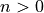, optional

The number of components.

valuefloat, optional

The components value. Default creates a null vector.

Examples

Create a Point

>>> import openturns as ot
>>> x = ot.Point(3, 1.0)
>>> x
class=Point name=Unnamed dimension=3 values=[1,1,1]


Get or set terms

>>> print(x)
1.0
>>> x = 0.0
>>> print(x)
0.0
>>> print(x[:2])
[0,1]


Create a Point from a flat (1d) array, list or tuple

>>> import numpy as np
>>> y = ot.Point((0.0, 1.0, 2.0))
>>> y = ot.Point(range(3))
>>> y = ot.Point(np.arange(3))


and back

>>> z = np.array(y)


>>> print(x + y)
[0,2,3]
>>> print(x - y)
[0,0,-1]


Multiplication, division with a scalar

>>> print(x * 3.0)
[0,3,3]
>>> print(x / 3.0)
[0,0.333333,0.333333]


Methods

 add(*args) Append a component (in-place). at(*args) Access to an element of the collection. Reset the collection to zero dimension. dot(rhs) Compute the scalar product. find(val) Find the index of a given value. Accessor to the object's name. Accessor to the vector's dimension. Accessor to the object's id. Accessor to the object's name. Accessor to the object's shadowed id. Accessor to the vector's dimension (or size). Accessor to the object's visibility state. Test if the object is named. Test if the object has a distinguishable name. Check if the components are in decreasing order. Tell if the collection is empty. Check if the components are in increasing order. Check if the components are in nonincreasing or nondecreasing order. Check if the components are in nondecreasing order. Check if the components are in nonincreasing order. Compute the Euclidean (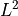) norm. Compute the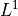norm. Compute the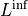norm. Compute the squared Euclidean norm. Compute the normalized vector with respect to its Euclidean norm. Compute the normalized vector with respect to its squared Euclidean norm. resize(newSize) Change the size of the collection. select(marginalIndices) Selection from indices. setName(name) Accessor to the object's name. Accessor to the object's shadowed id. setVisibility(visible) Accessor to the object's visibility state.
 clean
__init__(*args)

Append a component (in-place).

Parameters:
valuetype depends on the type of the collection.

The component to append.

Examples

>>> import openturns as ot
>>> x = ot.Point(2)
>>> print(x)
[0,0,1]

at(*args)

Parameters:
indexpositive int

Position of the element to access.

Returns:
elementtype depends on the type of the collection

Element of the collection at the position index.

clear()

Reset the collection to zero dimension.

Examples

>>> import openturns as ot
>>> x = ot.Point(2)
>>> x.clear()
>>> x
class=Point name=Unnamed dimension=0 values=[]

dot(rhs)

Compute the scalar product.

Parameters:
pointsequence of float

Scalar product second argument

Returns:
dotfloat

Scalar product

Examples

>>> import openturns as ot
>>> x = ot.Point([1.0, 2.0, 3.0])
>>> prod = x.dot([4, 5, 6])

find(val)

Find the index of a given value.

Parameters:
valcollection value type

The value to find

Returns:
indexint

The index of the first occurrence of the value, or the size of the container if not found. When several values match, only the first index is returned.

getClassName()

Accessor to the object’s name.

Returns:
class_namestr

The object class name (object.__class__.__name__).

getDimension()

Accessor to the vector’s dimension.

Returns:
nint

The number of components in the vector.

getId()

Accessor to the object’s id.

Returns:
idint

Internal unique identifier.

getName()

Accessor to the object’s name.

Returns:
namestr

The name of the object.

Accessor to the object’s shadowed id.

Returns:
idint

Internal unique identifier.

getSize()

Accessor to the vector’s dimension (or size).

Returns:
nint

The number of components in the vector.

getVisibility()

Accessor to the object’s visibility state.

Returns:
visiblebool

Visibility flag.

hasName()

Test if the object is named.

Returns:
hasNamebool

True if the name is not empty.

hasVisibleName()

Test if the object has a distinguishable name.

Returns:
hasVisibleNamebool

True if the name is not empty and not the default one.

isDecreasing()

Check if the components are in decreasing order.

Examples

>>> import openturns as ot
>>> x = ot.Point([3.0, 2.0, 1.0])
>>> x.isDecreasing()
True
>>> x = ot.Point([3.0, 3.0, 1.0])
>>> x.isDecreasing()
False
>>> x = ot.Point([1.0, 3.0, 2.0])
>>> x.isIncreasing()
False

isEmpty()

Tell if the collection is empty.

Returns:
isEmptybool

True if there is no element in the collection.

Examples

>>> import openturns as ot
>>> x = ot.Point(2)
>>> x.isEmpty()
False
>>> x.clear()
>>> x.isEmpty()
True

isIncreasing()

Check if the components are in increasing order.

Examples

>>> import openturns as ot
>>> x = ot.Point([1.0, 2.0, 3.0])
>>> x.isIncreasing()
True
>>> x = ot.Point([1.0, 1.0, 3.0])
>>> x.isIncreasing()
False
>>> x = ot.Point([1.0, 3.0, 2.0])
>>> x.isIncreasing()
False

isMonotonic()

Check if the components are in nonincreasing or nondecreasing order.

Examples

>>> import openturns as ot
>>> x = ot.Point([1.0, 2.0, 3.0])
>>> x.isMonotonic()
True
>>> x = ot.Point([2.0, 2.0, 1.0])
>>> x.isMonotonic()
True
>>> x = ot.Point([1.0, 3.0, 2.0])
>>> x.isMonotonic()
False

isNonDecreasing()

Check if the components are in nondecreasing order.

Examples

>>> import openturns as ot
>>> x = ot.Point([1.0, 2.0, 3.0])
>>> x.isNonDecreasing()
True
>>> x = ot.Point([1.0, 1.0, 3.0])
>>> x.isNonDecreasing()
True
>>> x = ot.Point([1.0, 3.0, 2.0])
>>> x.isNonDecreasing()
False

isNonIncreasing()

Check if the components are in nonincreasing order.

Examples

>>> import openturns as ot
>>> x = ot.Point([3.0, 2.0, 1.0])
>>> x.isNonIncreasing()
True
>>> x = ot.Point([3.0, 3.0, 1.0])
>>> x.isNonIncreasing()
True
>>> x = ot.Point([1.0, 3.0, 2.0])
>>> x.isNonIncreasing()
False

norm()

Compute the Euclidean () norm.

The Euclidean () norm of a vector is defined as: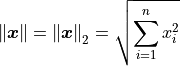Returns:
normfloat

The vector’s Euclidean norm.

Examples

>>> import openturns as ot
>>> x = ot.Point([1.0, 2.0, 3.0])
>>> x.norm()
3.741657...

norm1()

Compute thenorm.

Thenorm of a vector is defined as: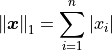Returns:
normfloat

The vector’snorm.

Examples

>>> import openturns as ot
>>> x = ot.Point([1.0, 2.0, 3.0])
>>> x.norm1()
6.0

normInf()

Compute thenorm.

Thenorm of a vector is defined as: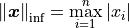Returns:
normfloat

The vector’snorm.

Examples

>>> import openturns as ot
>>> x = ot.Point([1.0, 2.0, 3.0])
>>> x.normInf()
3.0

normSquare()

Compute the squared Euclidean norm.

Returns:
normfloat

The vector’s squared Euclidean norm.

Examples

>>> import openturns as ot
>>> x = ot.Point([1.0, 2.0, 3.0])
>>> x.normSquare()
14.0

normalize()

Compute the normalized vector with respect to its Euclidean norm.

Returns:
normalized_vectorPoint

The normalized vector with respect to its Euclidean norm.

Raises:
RuntimeErrorIf the Euclidean norm is zero.

Examples

>>> import openturns as ot
>>> x = ot.Point([1.0, 2.0, 3.0])
>>> print(x.normalize())
[0.267261,0.534522,0.801784]

normalizeSquare()

Compute the normalized vector with respect to its squared Euclidean norm.

Returns:
normalized_vectornormalized_vectorPoint

The normalized vector with respect to its squared Euclidean norm.

Raises:
RuntimeErrorIf the squared Euclidean norm is zero.

Examples

>>> import openturns as ot
>>> x = ot.Point([1.0, 2.0, 3.0])
>>> print(x.normalizeSquare())
[0.0714286,0.285714,0.642857]

resize(newSize)

Change the size of the collection.

Parameters:
newSizepositive int

New size of the collection.

Notes

If the new size is smaller than the older one, the last elements are thrown away, else the new elements are set to the default value of the element type.

Examples

>>> import openturns as ot
>>> x = ot.Point(2, 4)
>>> print(x)
[4,4]
>>> x.resize(1)
>>> print(x)

>>> x.resize(4)
>>> print(x)
[4,0,0,0]

select(marginalIndices)

Selection from indices.

Parameters:
indicessequence of int

Indices to select

Returns:
collsequence

Sub-collection of values at the selection indices.

setName(name)

Accessor to the object’s name.

Parameters:
namestr

The name of the object.

Accessor to the object’s shadowed id.

Parameters:
idint

Internal unique identifier.

setVisibility(visible)

Accessor to the object’s visibility state.

Parameters:
visiblebool

Visibility flag.

## Examples using the class¶Randomize the lines of a Sample

Randomize the lines of a SampleA quick start guide to the Point and Sample classes

A quick start guide to the Point and Sample classesEstimate Wilks and empirical quantile

Estimate Wilks and empirical quantileEstimate correlation coefficients

Estimate correlation coefficientsCompare unconditional and conditional histograms

Compare unconditional and conditional histogramsCompute SRC indices confidence intervals

Compute SRC indices confidence intervalsEstimate a GEV on the Venice sea-levels data

Estimate a GEV on the Venice sea-levels dataBandwidth sensitivity in kernel smoothing

Bandwidth sensitivity in kernel smoothingFit a non parametric distribution

Fit a non parametric distributionEstimate a GEV on the Port Pirie sea-levels data

Estimate a GEV on the Port Pirie sea-levels dataEstimate a GEV on the Fremantle sea-levels data

Estimate a GEV on the Fremantle sea-levels dataEstimate a GEV on race times data

Estimate a GEV on race times dataKolmogorov-Smirnov : understand the statistics

Kolmogorov-Smirnov : understand the statisticsEstimate a scalar ARMA process

Estimate a scalar ARMA processExport a field to VTK

Export a field to VTKCreate a custom covariance model

Create a custom covariance modelDraw a field

Draw a fieldTrend computation

Trend computationOver-fitting and model selection

Over-fitting and model selectionCreate a polynomial chaos metamodel by integration on the cantilever beam

Create a polynomial chaos metamodel by integration on the cantilever beamCreate a polynomial chaos metamodel

Create a polynomial chaos metamodelCreate a polynomial chaos for the Ishigami function: a quick start guide to polynomial chaos

Create a polynomial chaos for the Ishigami function: a quick start guide to polynomial chaosCreate a sparse chaos by integration

Create a sparse chaos by integrationKriging: propagate uncertainties

Kriging: propagate uncertaintiesKriging : draw the likelihood

Kriging : draw the likelihoodExample of multi output Kriging on the fire satellite model

Example of multi output Kriging on the fire satellite modelKriging: metamodel of the Branin-Hoo function

Kriging: metamodel of the Branin-Hoo functionSequentially adding new points to a kriging

Sequentially adding new points to a krigingKriging :configure the optimization solver

Kriging :configure the optimization solverKriging : choose a trend vector space

Kriging : choose a trend vector spaceMetamodel of a field function

Metamodel of a field functionEvaluate the mean of a random vector by simulations

Evaluate the mean of a random vector by simulationsAnalyse the central tendency of a cantilever beam

Analyse the central tendency of a cantilever beamUse the post-analytical importance sampling algorithm

Use the post-analytical importance sampling algorithmEstimate a flooding probability

Estimate a flooding probabilityUse the Importance Sampling algorithm

Use the Importance Sampling algorithmExploitation of simulation algorithm results

Exploitation of simulation algorithm resultsUse the FORM algorithm in case of several design points

Use the FORM algorithm in case of several design pointsSubset Sampling

Subset SamplingTest the design point with the Strong Maximum Test

Test the design point with the Strong Maximum TestTime variant system reliability problem

Time variant system reliability problemAxial stressed beam : comparing different methods to estimate a probability

Axial stressed beam : comparing different methods to estimate a probabilityAn illustrated example of a FORM probability estimate

An illustrated example of a FORM probability estimateCross Entropy Importance Sampling

Cross Entropy Importance SamplingEstimate Sobol indices on a field to point function

Estimate Sobol indices on a field to point functionFAST sensitivity indices

FAST sensitivity indicesEstimate Sobol’ indices for a function with multivariate output

Estimate Sobol' indices for a function with multivariate outputUse the ANCOVA indices

Use the ANCOVA indicesThe HSIC sensitivity indices: the Ishigami model

The HSIC sensitivity indices: the Ishigami modelExample of sensitivity analyses on the wing weight model

Example of sensitivity analyses on the wing weight modelCreate mixed deterministic and probabilistic designs of experiments

Create mixed deterministic and probabilistic designs of experimentsCreate a composed function

Create a composed functionCreate an aggregated function

Create an aggregated functionCreate a linear combination of functions

Create a linear combination of functionsDefining Python and symbolic functions: a quick start introduction to functions

Defining Python and symbolic functions: a quick start introduction to functionsFunction manipulation

Function manipulationCalibrate a parametric model: a quick-start guide to calibration

Calibrate a parametric model: a quick-start guide to calibrationCalibration without observed inputs

Calibration without observed inputsCalibration of the logistic model

Calibration of the logistic modelCalibration of the deflection of a tube

Calibration of the deflection of a tubeCalibration of the flooding model

Calibration of the flooding modelCalibration of the Chaboche mechanical model

Calibration of the Chaboche mechanical modelSampling from an unnormalized probability density

Sampling from an unnormalized probability densityLinear Regression with interval-censored observations

Linear Regression with interval-censored observationsEstimate an integral

Estimate an integralCompute leave-one-out error of a polynomial chaos expansion

Compute leave-one-out error of a polynomial chaos expansionCompute confidence intervals of a regression model from data

Compute confidence intervals of a regression model from dataCompute confidence intervals of a univariate noisy function

Compute confidence intervals of a univariate noisy functionMix/max search and sensitivity from design

Mix/max search and sensitivity from designQuick start guide to optimization

Quick start guide to optimizationOptimization of the Rastrigin test function

Optimization of the Rastrigin test functionEfficientGlobalOptimization examples

EfficientGlobalOptimization examplesEstimate moments iteratively

Estimate moments iterativelyEstimate extrema iteratively

Estimate extrema iterativelyEstimate threshold exceedance iteratively

Estimate threshold exceedance iteratively